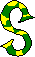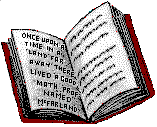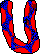TORY PROBLEMSnderstanding story problems is like understanding people: no single strategy works for all. Nevertheless, a few common themes can be found. The common themes are largely the result of the writing styles of text authors, so when you leave the classroom to solve problems in the outer world, expect far more variety than you see in our classroom! See general suggestions?

The following are examples of specific problem types
 Cost, Revenue, and Profit Compound Interest and Annuities Linear Programming (Math 143; no table) Linear Programming (Math 143; info in table) Conditional Probability (Math 143 Chp 7) Using Bayes Formula (Math 143 Chp 7, simpler problem) Using Bayes Formula (Math 143 Chp 7, harder problem) Rates of change (Calculus) Maximizing Revenue without calculus (Calc or Math 143) Maximizing Revenue with calculus (Calculus) Minimizing Area (Calculus) A box problem (Calculus) Minimizing cost of a box (Calculus) Albert's Pattern (Calc or Math 143)

GENERAL SUGGESTIONS FOR SOLVING TEXTBOOK STORY PROBLEMS

 Search the problem for the question (or demand), usually at the end.

"How many apples should Alice buy?"
"Find the number of apples Alice should buy."

  Invent variables to represent the answer to the question(s). It may be helpful to invent variables to represent other named quantities.

"Let x = the number of apples Alice should buy."

  Draw a picture of any physical features described in the problem, such as rectangles, paths, containers, or sets. If several quantities are mentioned, organize the information in a chart or table.

  (The hard step) Using your newly chosen variable(s), translate the English of the problem into mathematics, phrase by phrase, sentence by sentence. In our class, the mathematical statement will usually be an equation or inequality.

"3x+2=8"

 (The easy part, usually) Solve the mathematically expressed problem.

"x=2"

 Translate the mathematical solution back into English.

"Alice should buy 2 apples"